# AP Physics C Electricity : Understanding Capacitors

## Example Questions

### Example Question #1 : Capacitors

A parallel plate capacitor has a capacitance of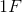. If the plates are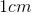apart, what is the area of the plates?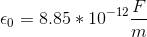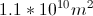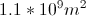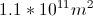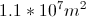Explanation:

The relationship between capacitance, distance, and area is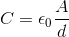. We can rearrange this equation to solve for area.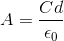Now, we can use the values given in teh question to solve.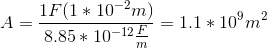### Example Question #1 : Capacitors

Charge is distributed uniformly over the area of the two plates of a parallel plate capacitor, resulting in a surface area charge density of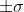on the plates (the top plate is positive and the bottom is negative, as shown below). Each plate has areaand are separated by distance. A material of dielectric constant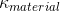has been placed between the two plates.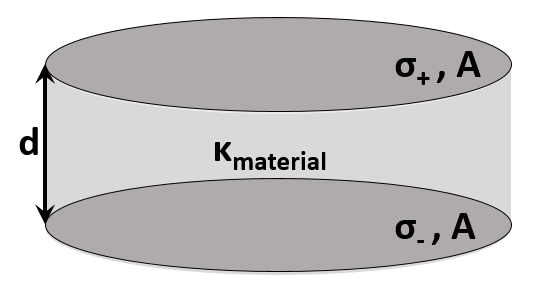Which of the following would not result in an increase in the measure of electric potential difference between the two plates?

Increase the distance,, between the plates

Increase the area,, of the plates

Replace the material between the two plates with one of a lower dielectric constant,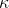Increase the value of the surface charge density,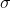, on each plate

Increase the area,, of the plates

Explanation:

The electric potential difference created between the plates of a parallel plate capactor is given by the equation: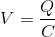The charge can be calculated by using the equation: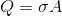The value of the capacitance is related to the dimensions of the capacitor with the equation: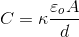Combining these equations yields: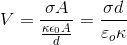The area becomes inconsequential, while the potential is directly proportional to the surface charge density and the distance between the plates, and inversely proportional to the dielectric of the material between the plates. Changing the area does not cause any change in the potential difference measured between the plates, and changing any of the other variables would cause a resultant change in the potential difference.